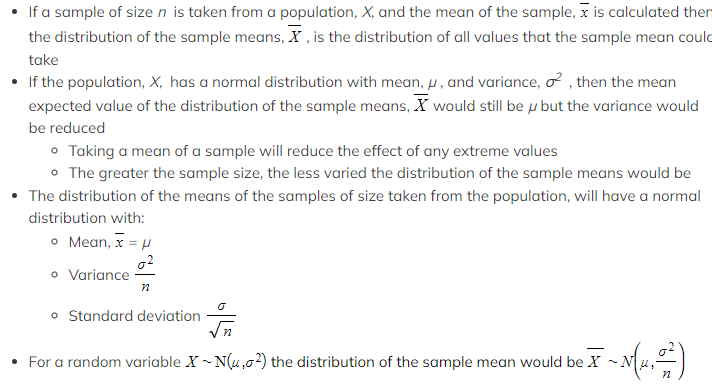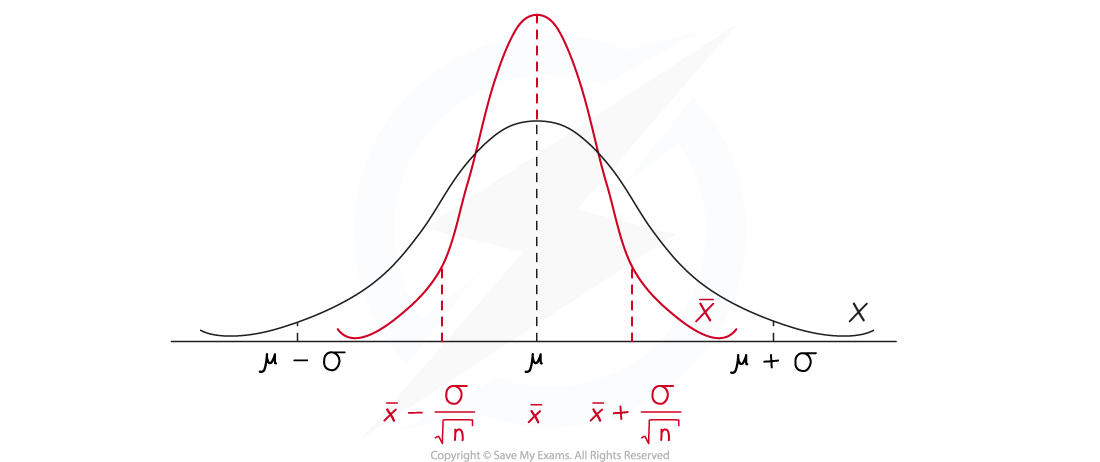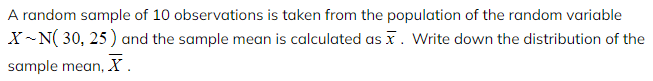# AQA A Level Maths: Statistics复习笔记5.3.1 Sample Mean Distribution

### Sample Mean Distribution

#### What is the distribution of the sample means?

• For any given population it can often be difficult or impractical to find the true value of the population mean, µ
• The population could be too large to collect data using a census or
• Collecting the data could compromise the individual data values and therefore taking a census could destroy the population
• Instead, the population mean can be estimated by taking the mean from a sample from within the population
•The standard deviation of the distribution of the sample means depends on the sample size, n
• It is inversely proportional to the square root of the sample size
• This means that the greater the sample size, the smaller the value of the standard deviation and the narrower the distribution of the sample means#### Worked Example#### Exam Tip

• Look carefully at the distribution given to determine whether the variance or the standard deviation has been given.